# Other non-repeating patterns

There are 134 remaining patterns which we divide into four cases below.

The first group are the nine Unique Factorization Domain patterns. If that does not mean anything to you, then consider the next group. An example of two of these are: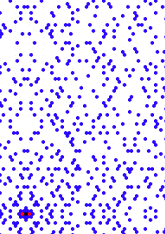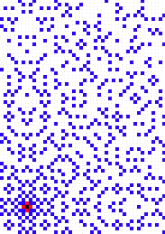For this group, of 9 patterns (6% percent of total), see page, or the paper
The second group are example of square divided into similar triangle. An example of two of these are: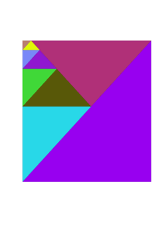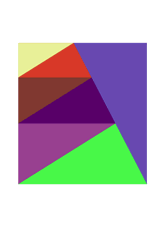For this group of 10 patterns (7% percent of total), see page. For a tabulation of their properties, see Triangles in a square.
The third group are examples of pattern in a strip. An example of two of these are: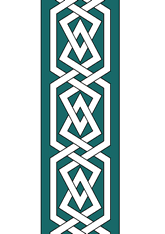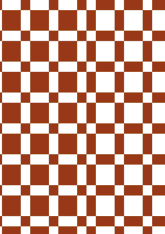In the second example, the central strip has a border on both sides. For this group, of 8 patterns (5% percent of total), see page.
The fourth group are examples of pattern with a centre and symmetry round that point. An example of two of these are: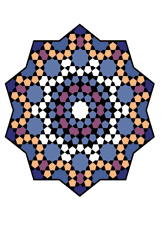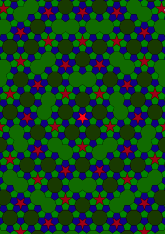For this group, of 104 patterns (77% percent of total), see the next choice page.
The last group are examples of patterns with no symmetry of any of the properties above. An example of two of these are: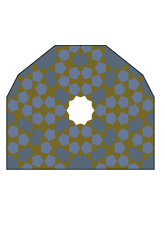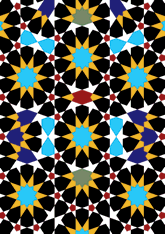For this group, of 3 patterns (2% percent of total), see page.

v53

Tiling Search was created by Brian Wichmann, and is maintained by MIT Libraries, through support from the Aga Khan Documentation Center.

Also supported by Performant Software Solutions LLC.